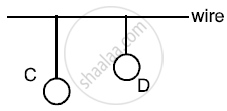# Two Pendulums C and D Are Suspended from a Wire as Shown in the Figure Give Below. Pendulum C is Made to Oscullate by Displaying It from Its Mean Position. - Physics

Short Note

Two pendulums C and D are suspended from a wire as shown in the figure give below. Pendulum C is made  to oscullate by displaying it from its mean position. It is seen that D also starts oscillating.(i) Name the type of oscillation, C will execute.
(ii) Name the type of oscillation, D will execute.
(iii) If the length of D is made equal to C then what difference will you notice in the oscillations of D ?
(iv) What is the name of the phenomenon when the length of D is made equal to C ?

#### Solution

(i) C will execute damped oscillation.
(ii) D will execute forced oscillation.
(iii) D will start executing with very large amplitude.
(iv) Resonance

Concept: Forced Vibrations
Is there an error in this question or solution?